Next: Motion with constant velocity Up: Motion in 3 dimensions Previous: Diagonals of a parallelogram

## Vector velocity and vector acceleration

Consider a body moving in 3 dimensions. Suppose that we know the Cartesian coordinates,,, and, of this body as time,, progresses. Let us consider how we can use this information to determine the body's instantaneous velocity and acceleration as functions of time.

The vector displacement of the body is given by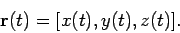(43)

By analogy with the 1-dimensional equation (13), the body's vector velocityis simply the derivative ofwith respect to. In other words,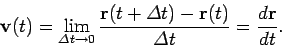(44)

When written in component form, the above definition yields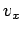(45)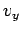(46)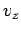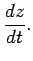(47)

Thus, the-component of velocity is simply the time derivative of the-coordinate, and so on.

By analogy with the 1-dimensional equation (16), the body's vector accelerationis simply the derivative of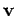with respect to. In other words,(48)

When written in component form, the above definition yields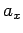(49)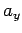(50)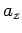(51)

Thus, the-component of acceleration is simply the time derivative of the-component of velocity, and so on.

As an example, suppose that the coordinates of the body are given by(52)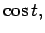(53)(54)

The corresponding components of the body's velocity are then simply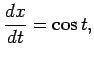(55)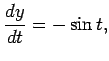(56)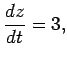(57)

whilst the components of the body's acceleration are given by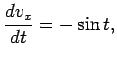(58)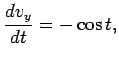(59)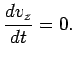(60)Next: Motion with constant velocity Up: Motion in 3 dimensions Previous: Diagonals of a parallelogram
Richard Fitzpatrick 2006-02-02